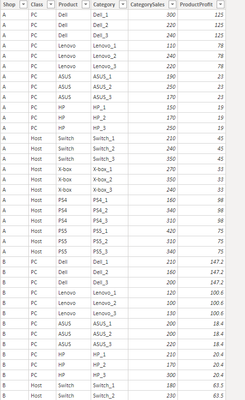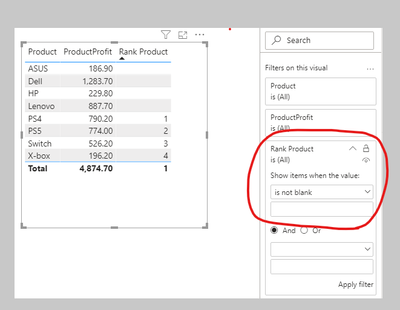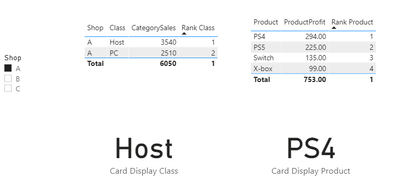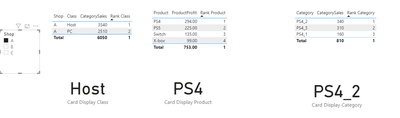cancel
Showing results for
Did you mean:## Ranking in 3 different items

Scenario:

Suppose I have a sales table including different categories with hierarchy, now I want to rank the sales and profits based on each hierarchy, how will I achieve this requirement?

This Blog is based on Function RANKX.

Table used:Shop B and Shop C in the sample table are similar with Shop A, so I won’t list them all here.

Guide Line:

1. We need to calculate the first rank hierarchy based on [Class] field.
2. According to the fist rank value, we need to filter the table which the first rank = 1 to calculate the second rank based on [Product] field.
3. Similar with the first rank value, we need to filter the table which the second rank = 1 to calculate the third rank based on [Category] field.

Detailed Steps:

1. The first step is to calculate the main hierarchy rank based on the Class column for each shop, we can create this measure like this:
``````Rank Class =
RANKX (
ALLSELECTED ( 'Table'[Class] ),
CALCULATE ( SUM ( 'Table'[CategorySales] ) ),
,
DESC,
DENSE
) ``````

1. Create another measure to show the first name of rank for Class in the card visual:
``````Card Display Class =
CALCULATE (
MAX ( 'Table'[Class] ),
FILTER ( ALLSELECTED ( 'Table'[Class] ), [Rank Class] = 1 )
) ``````

1. When we use a shop slicer to select shop A, it will give us the following result:1. Back to Step2, now we know the first rank of Class column is Host so we want a measure to calculate the rank based on the Host class without any other values. In this case, we can create a calculated table as variable to filter [Rank Class] = 1 and return a rank value like this:
``````Rank Product =
VAR a =
CALCULATETABLE (
FILTER ( DISTINCT ( 'Table'[Class] ), [Rank Class] = 1 ),
ALLSELECTED ()
)
RETURN
IF (
CALCULATE ( COUNTROWS ( 'Table' ), 'Table'[Class] IN a ) = 0,
BLANK (),
RANKX (
GROUPBY (
FILTER ( ALLSELECTED ( 'Table' ), 'Table'[Class] IN a ),
'Table'[Product]
),
CALCULATE ( SUM ( 'Table'[ProductProfit] ), 'Table'[Class] IN a ),
,
DESC,
DENSE
)
)

``````

1. Create another measure to show the first name of rank for Product in the card visual:
``````Card Display Product =
CALCULATE (
MAX ( 'Table'[Product] ),
FILTER ( ALLSELECTED ( 'Table'[Product] ), [Rank Product] = 1 )
) ``````

1. By the rank measure, if we put it in the table visual directly it will give us the rank value and the blank value at the same time so we need to set the measure value is not blank in the visual filter:This time when we use the shop slicer to select shop A, it will give us the following result:1. After the above two steps, we have achieved most of the requirements, now we need to get the final step which is almost the same as step2.

Requirement: Since the first rank of Product is PS4, we need to rank their categories.

Create the similar measure like step2:

``````Rank Category =
VAR a =
CALCULATETABLE (
FILTER ( DISTINCT ( 'Table'[Product] ), [Rank Product] = 1 ),
ALLSELECTED ()
)
RETURN
IF (
CALCULATE ( COUNTROWS ( 'Table' ), 'Table'[Product] IN a ) = 0,
BLANK (),
RANKX (
GROUPBY (
FILTER ( ALLSELECTED ( 'Table' ), 'Table'[Product] IN a ),
'Table'[Category]
),
CALCULATE ( SUM ( 'Table'[CategorySales] ), 'Table'[Product] IN a ),
,
DESC,
DENSE
)
) ``````

1. Create another measure to display the first name of rank for Category in card visual:
``````Card Display Category =
CALCULATE (
MAX ( 'Table'[Category] ),
FILTER ( ALLSELECTED ( 'Table'[Category] ), [Rank Category] = 1 )
) ``````

1. Set the rank measure value is not blank in the visual filter:After these steps, all the requirements are achieved, when we use the shop slicer to select Shop A, the whole result will be like this:This is how can we create hierarchy ranks in power bi by Dax. Hope this article helps everyone with similar questions here.

Author:  Yingjie Li

Reviewer: Ula Huang, Kerry Wang

Top Kudoed Posts
Latest Articles